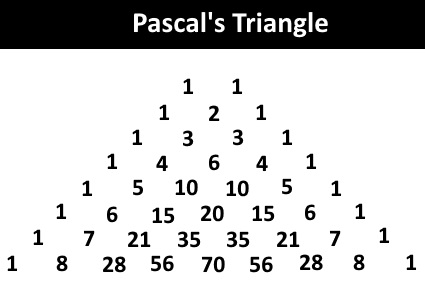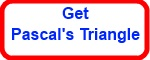# Mathematics

### The Binomial Expansion

This tutorial is developed in such a way that even a student with modest mathematics background can understand this particular topics in mathematics. It normally comes in core mathematics module 2 at AS Level.

Throughout the tutorial - and beyond it - students are discouraged from using the calculator in order to find nCr values. Instead, the use of Pascal's Triangle is encouraged, which is much faster than pressing down a series of calculator buttons.

#### Factorial - n!

The factorial of a number is defined as follows:

n! = n(n-1)n-2)(n-3).......1

E.g.

3! = 3 X 2 X 1 = 6

4! = 4 X 3 X 2 X 1 = 24

5! = 5 X 4 X 3 X 2 X 1 = 120

The factorial of both 0 and 1 are defined as 1 - 0! = 1; 1! = 1.

#### Factorial Calculator - n!

n

Now, let's deal with some simple calculations involving the factorials of numbers:

E.g.1

Find 5!/3!

5!/3! = 5 X 4 X 3!/3!
= 5 X 4
= 20
We stop the expansion of the top factorial at 3 so that the factorial of 3 at the bottom can be cancelled out.

E.g.2

Find 6!/4!

6!/4! = 6 X 5 X 4!/4!
= 6 X 5
= 30
We stop the expansion of the top factorial at 4 so that the factorial of 4 at the bottom can be cancelled out.

E.g.3

Find 6!/5!

6!/5! = 6 X 5!/5!
= 6
= 6
We stop the expansion of the top factorial at 5 so that the factorial of 5 at the bottom can be cancelled out.

E.g.4

Find 6!/(4! 2!)

6!/(4! 2!) = 6 X 5 X 4!/(4! 2!)
= 6 X 5 X 4!/ 4! 2!
= 30 / 2!
= 30/2
= 15 We stop the expansion of the top factorial at 4, the bigger factorial of the denominator, so that the factorial of 4 at the bottom can be cancelled out.

E.g.5

Find 6!/(5! 1!)

6!/(5! 1!) = 6 X 5!/(5! 1!)
= 6 X 5!/ 5! 1!
= 6 / 1!
= 6 / 1
= 6 We stop the expansion of the top factorial at 5, the bigger factorial of the denominator, so that the factorial of 5 at the bottom can be cancelled out.

E.g.6

Find 6!/(6! 0!)

6!/(6! 0!) = 6!/(6! 0!)
= 6!/ 6! 0!
= 1 / 0!
= 1 / 1
= 1 We stop the expansion of the top factorial at 6, the bigger factorial of the denominator, so that the factorial of 6 at the bottom can be cancelled out.

#### Combinations - nCr

nCr = n!/(n-r)!r!

E.g.1

Find 6C2.

6C2 = 6!/(6-2)!2!
= 6! / 4! 2!
= 6 X 5 X 4!/4! 2!
= 30 / 2!
= 30 / 2
= 15

E.g.2

Find 6C2.

7C5 = 7!/(7-5)!5!
= 7! / 2! 5!
= 7 X 6 X 5!/2! 5!
= 42 / 2!
= 30 / 2
= 21

E.g.3

Find 8C1.

8C1 = 8!/(8-1)!1!
= 8! / 7! 1!
= 8 X 7!/7! 1!
= 8 / 1!
= 8 / 1
= 8

E.g.4

Find 8C0.

8C0 = 8!/(8-0)!0!
= 8! / 8! 0!
= 8!/8! 0!
= 1 / 0!
= 1 / 1
= 1

#### Pascal's Triangle

Pascal's triangle gives all the nCr values of the nth row of the triangle.E.g.

For example, take the 6th row:
6C0 = 1
6C1 = 6
6C2 = 15
6C3 = 20
6C4 = 15
6C5 = 6
6C6 = 1

Now you can experiment with it using the calculator given below:

n:    r:

#### Formula for Binomial Expansion

(1 + x)n =Σ nCr xr

It is easier to get nCr values from Pascal's Triangle than from a calculator; typing takes time!

E.g.1

Expand (1 + x)5

(1 + x)5 = Σ 5Cr xr
= 5C0 x0 + 5C1 x1 + 5C2 x2 + 5C3 x3 + 5C4 x4 + 5C5 x5
= 1 + 5x + 10x2 + 10x3 + 5x4 + x5

E.g.2

Expand (1 + x)3

(1 + x)3 = Σ 3Cr xr
= 3C0 x0 + 3C1 x1 + 3C2 x2 + 3C3 x3
= 1 + 3x + 3x2 + x3

E.g.3

Expand (1 + 2x)5

(1 + 2x)5 = Σ 5Cr (2x)r
= 5C0 (2x)0 + 5C1 (2x)1 + 5C2 (2x)2 + 5C3 (2x)3 + 5C4 (2x)4 + 5C5 (2x)5
= 1 + 10x + 40x2 + 80x3 + 80x4 + 32x5

E.g.4

Expand (1 + x/2)5

(1 + x/2)5 = Σ 5Cr (x/2)r
= 5C0 (x/2)0 + 5C1 (x/2)1 + 5C2 (x/2)2 + 5C3 (x/2)3 + 5C4 (x/2)4 + 5C5 (x/2)5
= 1 + 5/2x + 5/2x2 + 5/4x3 + 5/16x4 + x5/32E.g.5

Expand (1 - x)5

(1 + (-x))5 = Σ 5Cr (-x)r
= 5C0 (-x)0 + 5C1 (-x)1 + 5C2 (-x)2 + 5C3 (-x)3 + 5C4 (-x)4 + 5C5 (-x)5
= 1 - 5x + 10x2 - 10x3 + 5x4 - x5

E.g.6

Expand (2 + x)5

Take 2 out in order to perform binomial expansion
[2(1 + x/2)]5 =25[ Σ 5Cr (x/2) ]r
= 25[5C0 (x/2)0 + 5C1 (x/2)1 + 5C2 (x/2)2 + 5C3 (x/2)3 + 5C4 (x/2)4 + 5C5 (x/2)5]
= 25[1 + 5/2 x + 5/2 x2 + 5/4 x3 + 5/16 x4 + x5/32]
= 32 + 80x + 80x2 + 40x3 + 10x4 + x5

E.g.7

Find the coefficient of the third term of (3 + 2x)5

[3(1 + 2x/3)]5 =35[ Σ 5Cr (2x/3) ]r
The third term is 35 X 5C2 X (2x/3)2
35 X 10 X 4x2/32
33 X 10 X 4x2
1080x2
So, the coefficient of the 3rd term is 1080.

E.g.8

Show that nCr = nC(n-r)
nCr = n!/(n-r)! r! = n(n-1)(n-2)...(n-r+1)(n-r)!/(n-r)! r! = n(n-1)(n-2)...(n-r+1)/r!
nC(n-r)! = n!/(n-r)! r! = n(n-1)(n-2)...(n-r+1)(n-r)!/(n-(n-r))!(n-r)! = n(n-1)(n-2)...(n-r+1)(n-r)!/r!(n-r)!= n(n-1)(n-2)...(n-r+1)/r!
nCr = nC(n-r)

#### The Binomial Expansion - negative indices and fractions

(1 + x)n = Σ nCr
nC0 x0 + nC1 x + nC2 x2 + nC3 x3 + ......+ nCn xn
1 + nx + n(n-1)!/2!(n-2)! x2 + n(n-1)(n-2)(n-3)!/3! x3 +.......
1 + nx + n(n-1)/2 x2 + n(n-1)(n-2)/6 x3 +......
if x is very small - |x|<1 or -1< x <1 - the higher powers of x are getting too small and become insignificant.
So, the expansion can be stopped as follows:

#### (1 + x)n ≈ 1 + nx + n(n-1)/2 x2 + n(n-1)(n-2)/6 x3

Note the almost equal sign, instead of the equal sign.

E.g.1

Expand (1 + x)1/2 up to the fourth term.
(1 + x)n ≈ 1 + nx + n(n-1)/2 x2 + n(n-1)(n-2)/6 x3
(1 + x)1/2 ≈ 1 + (1/2)x + (1/2)(-1/2)/2 x2 + (1/2)(-1/2)(1/2-2)/6 x3
(1 + x)1/2 ≈ 1 + (1/2)x - (1/4)/2 x2 + (3/16)/6 x3
(1 + x)1/2 ≈ 1 + (1/2)x - (1/8) x2 - (1/16) x3

E.g.2

(1 + x)n ≈ 1 + nx + n(n-1)/2 x2 + n(n-1)(n-2)/6 x3
(1+x)−3 ≈ 1+(−3) x + (−3)(−4)/2! + x2 + (−3)(−4)(−5)/3! x3
(1+x)−3 ≈ 1 −3x + 6x2 − 10x3

E.g.3

Expand (1 + 2x)-3 and state the value/s of x for which the expansion is valid.
(1 + x)n ≈ 1 + nx + n(n-1)/2 x2 + n(n-1)(n-2)/6 x3
(1 + 2x)-3 ≈ 1 + (−3)(2x) + (−3)(−4)(2x )2/2! +(−3)(−4)(−5)(2x)3/3!
≈ 1 − 6x + 24x2 − 80x3
The expansion is valid if |2x| < 1
|x| <1/2 or -1/2 < x < 1/2

E.g.4

Expand (1 - x)-5 and state the value/s of x for which the expansion is valid.
(1 - x)n ≈ 1 + nx + n(n-1)/2 x2 + n(n-1)(n-2)/6 x3
(1 - x)-5 ≈ 1 + (−5)(-x) + (−5)(−6)(-x )2/2! +(−5)(−6)(−7)(-x)3/3!
≈ 1 + 5x + 15x2 + 35x3
The expansion is valid if |-x| < 1
|x| < 1 or -1 < x < 1

E.g.5

Expand (1 + 3x)1/2 and hence find an approximation for √103.
(1 + x)n ≈ 1 + nx + n(n-1)/2 x2 + n(n-1)(n-2)/6 x3
(1 + 3x)1/2 ≈ 1 + (1/2)(3x) + (1/2)(1/2-1)(3x)2/2! +(1/2)(1/2-1)(1/2-2)(3x)3/3!
≈ 1 + (3/2)x + (-9/8)x2 − (27/16)x3
Let x = 0.01
Then, (1 + 3 X 0.01)1/2 ≈ 1 + (3/2)0.01 + (-9/8)0.012 − (27/16)0.013
(1 + 3 X 0.01)1/2 ≈ 1 + (0.03/2) + (-0.0009/8) − (0.000027/16)
(1 + .03)1/2 ≈ 1 + 0.015 -0.0001125 − 0.0000017
(1 + 0.03)1/2 ≈ 1.0148858
(1 + 3/100)1/2 ≈ 1.0148858
(103/100)1/2 ≈ 1.0148858
1031/2/10 ≈ 1.0148858
√103 ≈ 10.148858
√103 ≈ 10.149(3 d.p)

Do you need the tutorial with more worked examples as a Kindle Book? Here is the link for Amazon:Maths is challenging; so is finding the right book. K A Stroud, in this book, cleverly managed to make all the major topics crystal clear with plenty of examples; popularity of the book speak for itself - 7th edition in print.

### Recommended - GCSE & iGCSEThis is the best book available for the new GCSE(9-1) specification and iGCSE: there are plenty of worked examples; a really good collection of problems for practising; every single topic is adequately covered; the topics are organized in a logical order.

### Recommended for A LevelThis is the best book that can be recommended for the new A Level - Edexcel board: it covers every single topic in detail;lots of worked examples; ample problems for practising; beautifully and clearly presented.